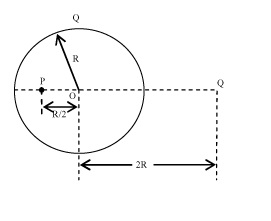# Electric field by chargesA point charge $Q$ is placed at a distance $2R$ from the centre of a conducting charged spherical shell of radius $R$ and having a charge $Q$ as shown. The electric potential at point $P$ due to charge on shell is ${\dfrac{aQ}{b \pi \epsilon_{o} R}}$, where $a$ and $b$ are coprime positive integers. Find $a+b$.

×